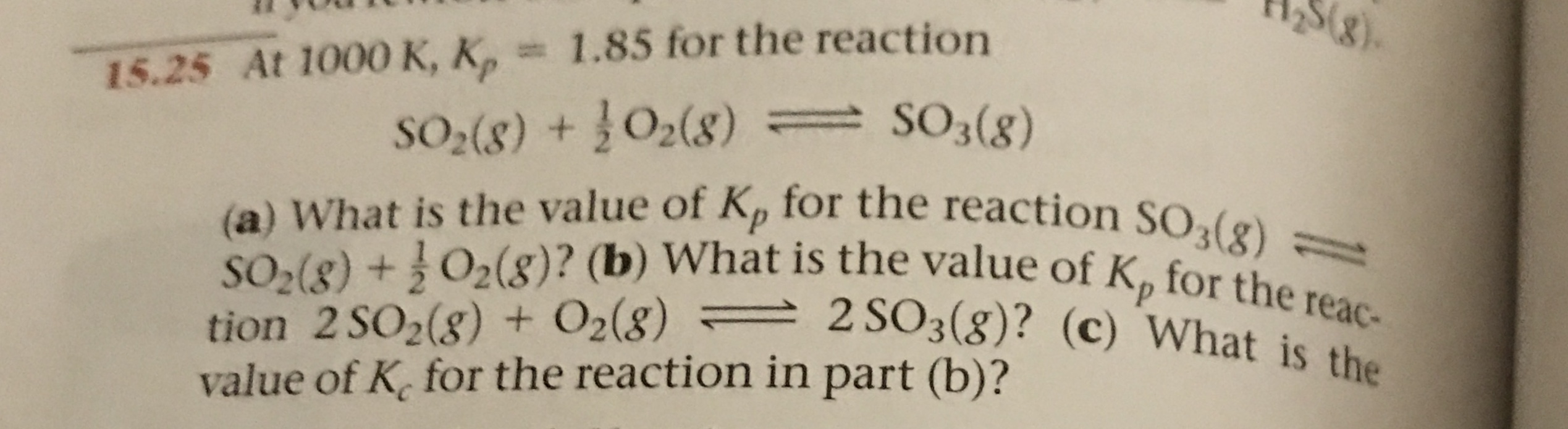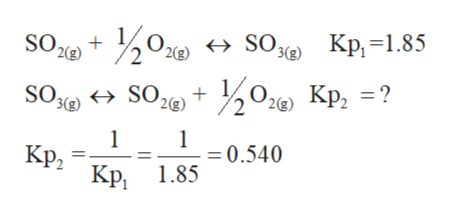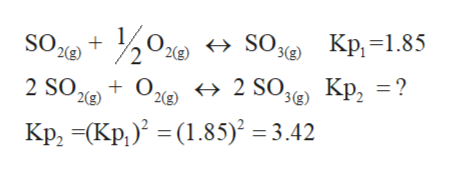# 258)15.25 At 1000 K, K, 1.85 for the reactionS2(8) +O2(8) SO3(8)(a) What is the value of Kp for the reaction SO3(8)-tion 2 SO2(g) + O2(8) 2 SO3(g)? (c) What is thevalue of K for the reaction in part (b)?SO2(8) +O2(8)? (b) What is the value of Kp for the reac-

Question
2 viewshelp_outlineImage Transcriptionclose258) 15.25 At 1000 K, K, 1.85 for the reaction S2(8) +O2(8) SO3(8) (a) What is the value of Kp for the reaction SO3(8)- tion 2 SO2(g) + O2(8) 2 SO3(g)? (c) What is the value of K for the reaction in part (b)? SO2(8) +O2(8)? (b) What is the value of Kp for the reac- fullscreen
check_circle

Step 1

A reversible chemical reaction can move in either forward or backward direction. The stage of a reversible reaction at which the rate of forward and backward reaction become equal to each other is called an equilibrium stage.

The equilibrium constant for a reaction is the ratio of equilibrium concentration of product and reactant. If the reaction is given in gaseous state, then the equilibrium pressure must be considered.

Step 2

Part (a)

The Kp value is given for the formation of SO3 at 1000 K as 1.85. For the reaction of conversion of SO3 to SO2 must be backward reaction of the formation of SO3.

Hence the Kp must be inverse value:help_outlineImage Transcriptionclose,0ло SO. SO2 → SO3 Kp,=1.85 2(g) 2(g) 3(g) SO26) + 50 д6) Кр, 3D? SOgo 2(3) Kp, Kp, 1.85 = 0.540 fullscreen
Step 3

Part (b):

The given reaction is just double to the reaction of formation o...help_outlineImage Transcriptionclose50до у SO, 2 SO23) SO, Kp, =1.85 2(g) 2(g) 3(g) + Oxe + 2 SO Kp, =? 2(g) 2(g) 3(g) Kp, =(Kp,) = (1.85)² = 3.42 fullscreen

### Want to see the full answer?

See Solution

#### Want to see this answer and more?

Solutions are written by subject experts who are available 24/7. Questions are typically answered within 1 hour.*

See Solution
*Response times may vary by subject and question.
Tagged in

### Chemistry